# SSAT Upper Level Math : How to find the length of the hypotenuse of a right triangle : Pythagorean Theorem

## Example Questions

### Example Question #1 : How To Find The Length Of The Hypotenuse Of A Right Triangle : Pythagorean Theorem

The base and height of a right triangle are each 1 inch. What is the hypotenuse?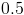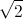Explanation:

You need to use the Pythagorean Theorem, which is.

Add the first two values and you get. Take the square root of both sides and you get.

### Example Question #1 : Apply The Pythagorean Theorem To Find The Distance Between Two Points In A Coordinate System: Ccss.Math.Content.8.G.B.8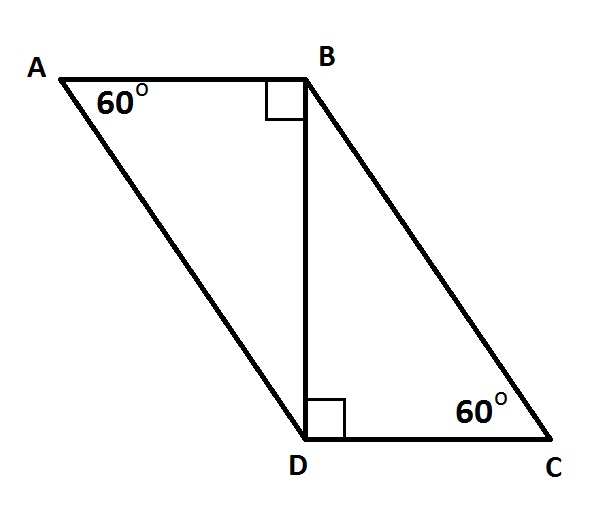Give the perimeter of the above parallelogram if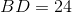.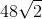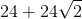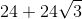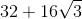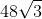Explanation:

By the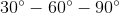Theorem: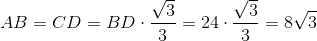, and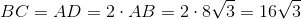The perimeter of the parallelogram is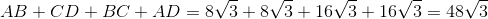### Example Question #2 : How To Find The Length Of The Hypotenuse Of A Right Triangle : Pythagorean Theorem

A right triangle has legs with lengths ofunits andunits. What is the length of the hypotenuse?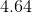unitsunits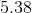units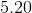unitsunits

Explanation: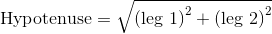Using the numbers given to us by the question,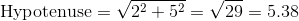units

### Example Question #3 : How To Find The Length Of The Hypotenuse Of A Right Triangle : Pythagorean Theorem

A right triangle has legs with the lengths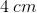and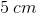. Find the length of the hypotenuse.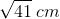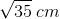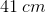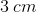Explanation:

Use the Pythagorean Theorem to find the length of the hypotenuse.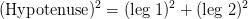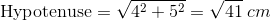### Example Question #4 : How To Find The Length Of The Hypotenuse Of A Right Triangle : Pythagorean Theorem

Find the length of the hypotenuse in the right triangle below.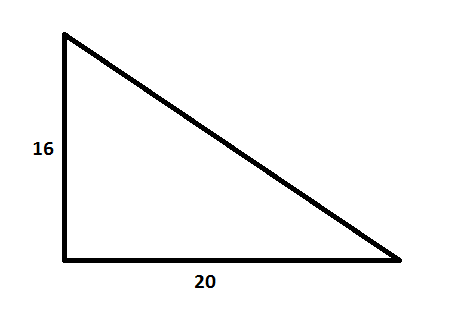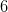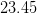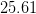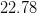Explanation:

Use the Pythagorean Theorem to find the hypotenuse.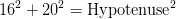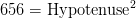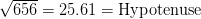### Example Question #5 : How To Find The Length Of The Hypotenuse Of A Right Triangle : Pythagorean Theorem

If James traveled north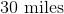and John traveled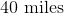west from the same town, how many miles away will they be from each other when they reach their destinations?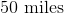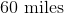Explanation:

The distances when put together create a right triangle.

The distance between them will be the hypotenuse or the diagonal side.

You use Pythagorean Theorem orto find the length.

So you plugandforandwhich gives you,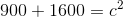or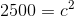.

Then you find the square root of each side and that gives you your answer of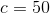.